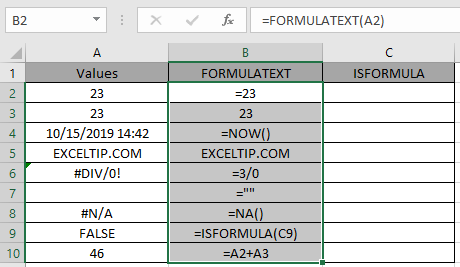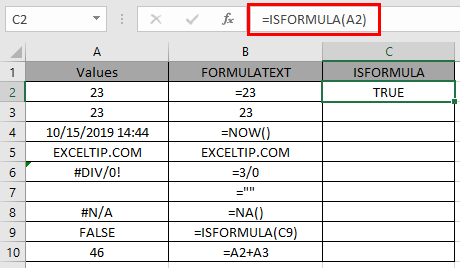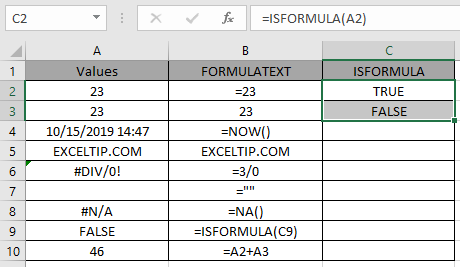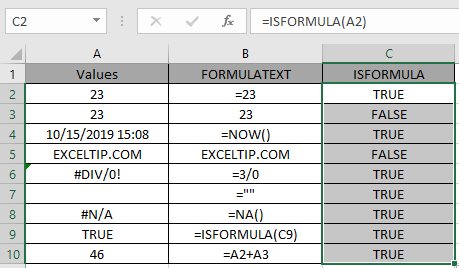# How to use the ISFORMULA Function in Microsoft Excel

FORMULA values in cell is the value returned by the formula. In Excel when there is a Formula in the cell, it starts with equals ( = ) sign. ISFORMULA function returns TRUE if the cell has a formula which returns output or else False. Sometimes an empty cell is output of a function which returns an empty string.

FORMULATEXT function is generally used to view the formula of a cell into another cell.
ISFORMULA function checks the value in the reference cell for a formula and returns TRUE if exist or else FALSE.
Syntax:

 = ISFORMULA ( reference )

reference : reference to a cell or range of cells.
Now let’s understand the function more via using it in an example.Here we have a list of output value in Values column and in FORMULATEXT column we have the input values which returns the output values in Values Column.
FORMULATEXT function is generally used to view the formula of a cell into another cell.
We will check the Values column if it has a formula or not
Use the formula:

 = ISFORMULA ( A2 )

A2 : reference cell is provided to the function to check its value.The function returns TRUE i.e. The value in the cell is result of a formula.
And now the result of A3 cell.You can observe from the above snapshots that there is a difference between direct input value and return output value. In A2 cell there is  number which is returned by a formula ( = 23 ) whereas in A3 cell, the number is the value of the cell.
Copy the formula to other cells using the Ctrl + D shortcut key.As you can see, the function returns the logic values either TRUE or FALSE. Below are some of the observational results.
Note:

1. Empty cell can have a formula in the cell. The formula returns an empty string in the cell
2. When range of cells given in as argument, the function only checks the first cell of the array as returns TRUE or FALSE based only on the first cell.
3. The function returns the  error if the reference to the function is invalid.

You can use the FORMULATEXT function to view the input value by user in the cell.

Use the formula:

 = FORMULATEXT ( reference )

Hope you understood how to use ISFORMULA function in Excel 2016. Find more articles on Excel ISLOGICAL function here. Please share your query below in the comment box. We will assist you.

Terms and Conditions of use

The applications/code on this site are distributed as is and without warranties or liability. In no event shall the owner of the copyrights, or the authors of the applications/code be liable for any loss of profit, any problems or any damage resulting from the use or evaluation of the applications/code.